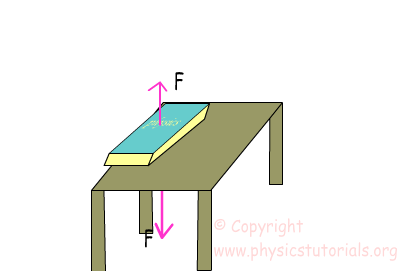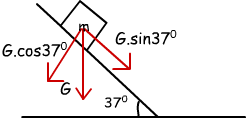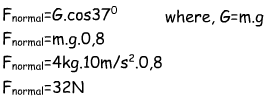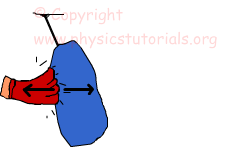# Normal Force with Examples

Normal Force:

Contact objects exert force to each other because of their weights. In this example, book exerts a force to table because of its weight and table also exerts force to the book. We call this force as “normal force” which is same in magnitude andopposite in direction with the applied force (weight of the book). For different situations, we say that in general normal force is the reaction to the perpendicular force exerting on it. We will deal with different examples of normal force. Look at the given examples below and follow the steps to understand how can we find normal force for different situations.

Example Find the normal force that the inclined plane exerts on the box. (sin37º=0, 6 cos37º=0, 8), (m=4kg, g=10m/s²)Since our box is on inclined plane we can not say directly the normal force is equal to the weight of the object. Normal force is equal to the force exerting on it perpendicularly. Let’s draw a free body diagram and show all the forces.Red arrows show the force of weight and its components. Horizontal and vertical components of force are found by using trigonometry. We said that for the normal force, there must be perpendicular force acting to the contact surface, thus, here the cause of normal force is the vertical component of the weight. Let’s calculate the value of it.Look at the given pictures. In the first picture a boy kicks the ball and exerts a force to the ball, to reaction this force ball also exerts a force to the foot. In the second picture again the forces are shown with arrows. You should be careful while drawing these arrows. Most of the students have difficulty in this point. Be careful, forces are acted on different objects, thus, we should show them on differentbodies. This explanation also answers the frequently asked question “If the forces are equal in magnitude and opposite in direction why they do not cancel each other”. From the explanation given above we say that,  they do not cancel each other because they are acting on different bodies or systems. Forces should be on the same object or system to cancel each other.

Dynamics Exams

Related